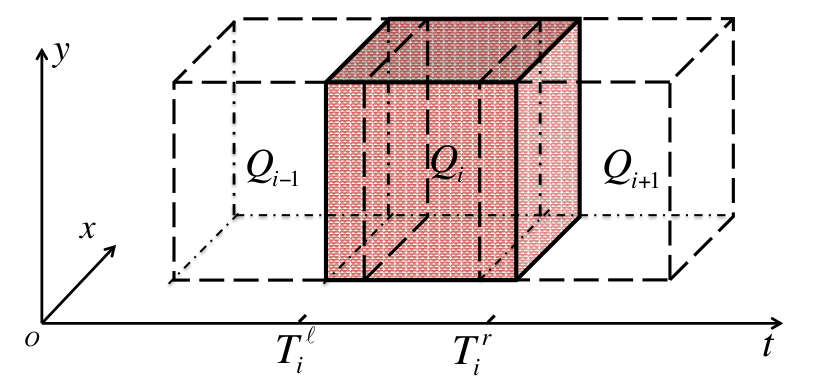# Teaching

2019 Fall: Engineering Mathematics, Differential Equations

2019 Spring: Calculus II, Partial Differential Equations

2018 Fall: Differential Equations, Theory of Ordinary Differential Equations

2018 Summer: MATH-567 Numerical Methods for PDEs

2018 Spring: Calculus II, Linear Algebra, Partial Differential Equations

2017 Fall: Engineering Numerical Analysis

Taught Courses at Jackson State University:

2017 Spring: Numerical Analysis, Ordinary Differential Equations

2016 Fall: Calculus III, Real Analysis (Grad)

2016 Summer: Business Calculus I

2016 Spring: Calculus II, Numerical Methods I (Grad)

2015 Fall: Calculus I, Numerical Methods II (Grad)

Taught Courses at Southern Illinois University, Carbondale:

2012 Fall: College Algebra

2012 Spring: Intro to Contemporary Math. (2 sessions)

2011 Fall: Intro to Contemporary Math. (3 sessions)

# Recent Publications

• A Modified Transient Technique for Porosity and Permeability Determination in Tight Formations: Experimental Investigation and Numerical Simulation

• Efficient Adaptive Qualitative Methods for 3D Inverse Scattering Problems

• Non-commutative Discretize-then-Optimize Algorithms for Elliptic PDE-Constrained Optimal Control Problems

• Two direct factorization methods for inverse scattering problems

• Effect of gas compressibility on permeability measurement in coalbed methane formations: Experimental investigation and flow modeling

# Research Projects#### Domain Decomposition

Develop Domain Decomposition Algorithms for PDE-Constrained Optimization

# Developed Codes

### MATLAB code for 1D Multigrid algorithm

Multigrid (MG) methods belong to the best known algorithms for solving some class of PDEs.This code gives a MATLAB implementation of 1D Multigrid algorithm for solving a two-point ODE boundary value problem. Compared with existing online codes, my code tries to balance well between efficiency and readability (simplicity) for practical and wide use.